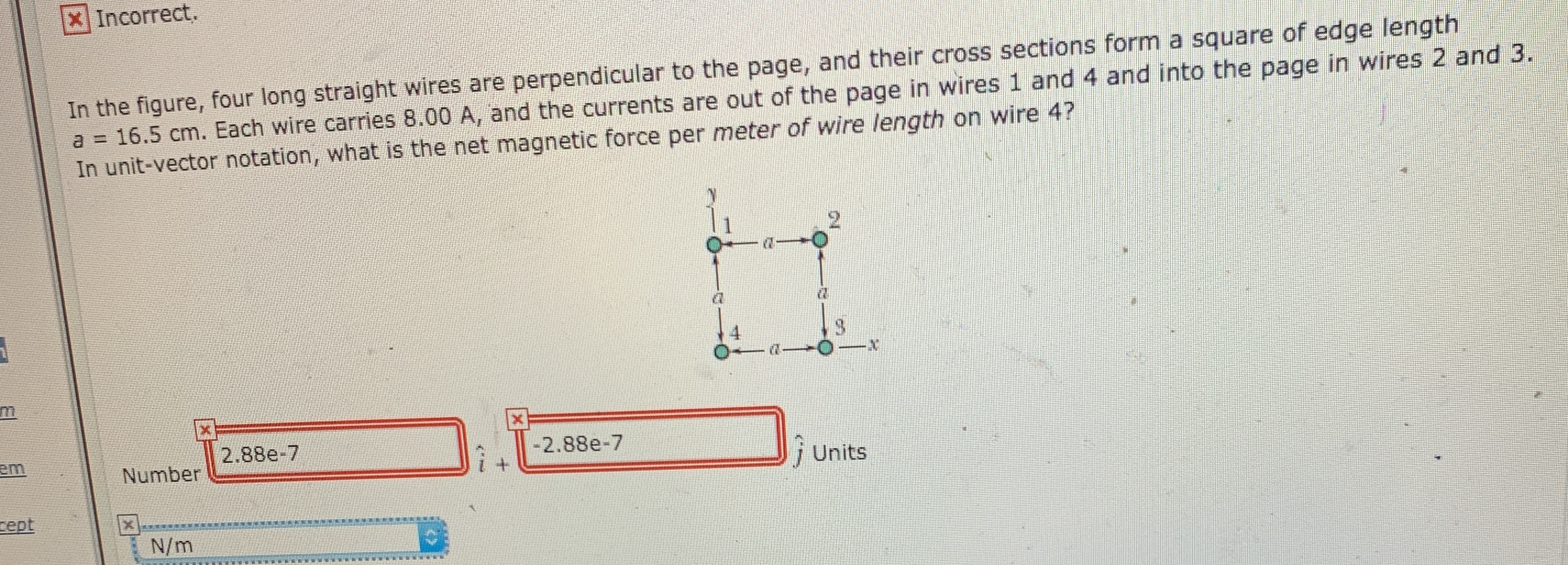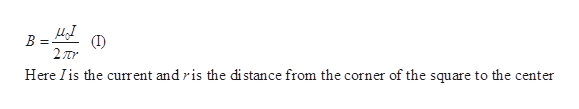# X Incorrect.In the figure, four long straight wires are perpendicular to the page, and their cross sections form a square of edge lengtha 16.5 cm. Each wire carries 8.00 A, and the currents are out of the page in wires 1 and 4 and into the page in wires 2 and 3.In unit-vector notation, what is the net magnetic force per meter of wire length on wire 4?2.88e-7-2.88e-7emi UnitsNumberteptXN/m

Questionhelp_outlineImage TranscriptioncloseX Incorrect. In the figure, four long straight wires are perpendicular to the page, and their cross sections form a square of edge length a 16.5 cm. Each wire carries 8.00 A, and the currents are out of the page in wires 1 and 4 and into the page in wires 2 and 3. In unit-vector notation, what is the net magnetic force per meter of wire length on wire 4? 2.88e-7 -2.88e-7 em i Units Number tept X N/m fullscreen
check_circle

Step 1

Each wire produces a field with magnitude given by,help_outlineImage TranscriptioncloseВ 2Tr Here Iis the current and ris the distance from the corner of the square to the center fullscreen
Step 2

By Pythagoras theorem, the diagonal of the square has the length a√2.

Then, r= (a√2)./2

Therefore,

Step 3

The magnetic field along all the wires will be the same

That is, B1=B2=B3=B4=B

Substitute th...

### Want to see the full answer?

See Solution

#### Want to see this answer and more?

Solutions are written by subject experts who are available 24/7. Questions are typically answered within 1 hour.*

See Solution
*Response times may vary by subject and question.
Tagged in

### Magnetic Effects of Current